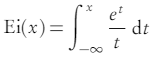# Entire Function: Simple Definition

## What is an Entire Function?

An entire function is analytic on the entire complex plane. It’s called an “entire” function because of this very fact.

This simple definition leads to a big problem when dealing with entire functions: The space of (set of all) entire functions is huge; So huge in fact, that it’s usually necessary to work with smaller families of maps to ensure strong results. A whole subset of complex analysis, called entire function theory, is devoted to the study of these useful functions.

The entire function is sometimes called the integral function. However, this can get a bit confusing because the term “integral function” also refers to an antiderivative for any function.

## Examples of Entire Functions

Some of the simplest entire functions are the exponential functions, polynomial functions (as long as the functions are complex-valued), and any finite compositions, products or sums of those two types.

A few specific examples of entire functions:

• ez
• zn
• sin(z)

Many of the simpler entire functions behave in a similar way, dynamically speaking, to polynomial functions. These include λ ez and acos z + b (Eremenko & Lyubich, 1992).

The natural logarithm function and the square root function are not analytic across the entire complex plane, so they are not entire functions.

## Special Classes of Entire Functions

• Speiser class (S) only have a finite number of singular values.
• Eremenko-Lyubich class functions of bounded type (B) are where all singular values are contained in a bounded set in ℂ.

## What is the Exponential Integral Function?

The exponential integral function is a special function used in astrophysics, quantum chemistry and many other applied sciences.

It can be defined in two different ways: as a real-valued function or as a complex-valued function.

## 1. Real-Valued Exponential Integral Function

The exponential integral function is defined as a definite integral, with a ratio of the exponential function and its argument:The function is defined for x in the set of natural numbers. This set excludes zero.

Or, equivalently the function can be defined a little differently through a parity transformation. A parity transformation is where the signs are flipped: t→ -t and x→ -x. This gives what Enrico Masina calls a “more suitable” definition:Note though, that despite the stated suitability of the above form, most authors use the notation E1 only for the complex-valued version of the function.

## 2. Exponential Integral Function Defined on the Complex Plane

On the complex plane, the function E1is also a definite integral.The notation is almost the same, with a couple of notable differences:

• The substitution of “z” (to denote a complex number) instead of “x”.
• While the “real” version of the function above is defined for the set of whole numbers, the complex-numbered version is defined for |arg(z) < π)|.*

*This comes from the exponential form of a complex number, z = |z|e, which is just z = x + i y rewritten with exponentials; θ = arg(z) = arctan(y/x).

The complex valued version is not valid when z = 0 or z = ∞, because of a specific kind of discontinuity called a branch point.

## References

Eremenk, A. & Lyubich, M. (1992). Dynamical properties of some classes of entire functions. Retrieved December 8, 2019 from: http://www.math.stonybrook.edu/~bishop/classes/math627.S13/EL-Fourier.pdf
Gardner, R. Zeros of an Analytic Function. Retrieved December 9, 2019 from: https://faculty.etsu.edu/gardnerr/5510/notes/IV-3.pdf
Knopp, K. (1996). Entire Transcendental Functions. Ch. 9 in Theory of Functions Parts I and II, Two Volumes Bound as One, Part I. New York: Dover, pp. 112-116, 1996.
Masina, E. (2017). A review on the Exponential-Integral special function and other strictly related special functions. Lectures from a seminar of Mathematical Physics. Retrieved December 10, 2019 from: https://www.researchgate.net/publication/323772322_A_review_on_the_Exponential-Integral_special_function_and_other_strictly_related_special_functions_Lectures_from_a_seminar_of_Mathematical_Physics
Orloff, J. Analytic Functions. Retrieved December 8, 2019 from: https://math.mit.edu/~jorloff/18.04/notes/topic2.pdf
Schleicher,, D. Dynamics of Entire Functions. Retrieved December 8, 2019 from: http://www.math.stonybrook.edu/~bishop/classes/math627.S13/DynamicsEntireOverview.pdf

CITE THIS AS:
Stephanie Glen. "Entire Function: Simple Definition" From StatisticsHowTo.com: Elementary Statistics for the rest of us! https://www.statisticshowto.com/entire-function-integral/